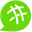# Day 1: Time Complexity

Hello All,
this is karthi and i'm gonna learn programming from today onwards so i start with interviewbit programming basics it's start with time complexity so i'm gonna use these platform as a journal for my learning process. let's see how it goes :) Peace!
Friendly Note: I have a very little knowledge in programming so if you think i'm going in a wrong way kindly let me know it will be much helpful!.

While running the program it is important to know how much time it takes to run and how much space it used from memory. the lower the time and space consumes the better result we can get from it.

There are three cases in Time Complexity:
1.Best Case(Ω)
2.Average Case(θ)
3.Worst Case(O)
Ex: 1 2 3 4 5 the 1 is best case and 3 is average case and 5 is the worst case result.
How to calculate time complexity?
I've seen these type of question in many interviews.. they given some program and ask us to calculate the time complexity of that program.... other type is write the program for a problem with given time complexity. so its important for us to know how to do it.
Example 1:
int a = 10; //1 unit
int 6 = 20; // 1 unit
sum = a+b; // 1 unit
so the time complexity of the problem is O(3).

Example 2:
for(i=0;i<=n;i++){
print(i);
}
for(i=0;i<=n;i++){
print(j);
}
In these problem n value is not provided so time complexity of the first and second for loop is O(n) and O(n) we can't write O(2n) for these as the loops run separately so the time complexity of these problem is O(n).

Example 3:
for(i=0li<=n;i++){
for(j=0;j<=n;j++){
print(i,j);
}}
In these nested loop we have to multiply n so the time complexity for these is O(n²) .
I hope i'm going correct even if there is some mistakes ill rectify it and correct it in future so that's it for today. Bye All

## Discussion (1)Comment deleted